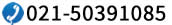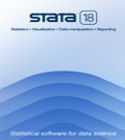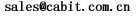Fixed- and random-effects models

• Linear model with panel-level effects and i.i.d. errors
• Linear model with panel-level effects and AR(1) errors
• GLS and ML estimators
• Robust and cluster-robust standard errors

Specification tests

• Hausman specification test
• Breusch and Pagan Lagrange multiplier test for random effects

Linear dynamic panel-data estimators

• Arellano–Bond estimator
• Arellano–Bover/Blundell–Bond system
• Opening, closing, and embedded gaps
• Serially correlated disturbances
• Complete control over instrument list
• Predetermined variables
• Tests for autocorrelation and of overidentifying restrictions

Panel-corrected standard errors (PCSE) for linear cross-sectional models

Two-stage least-squares panel-data estimators

• Between-2SLS estimator
• Within-2SLS estimator
• Baltagi EC2SLS estimator
• All with balanced or exogenously balanced panels

Multilevel mixed-effects models

Stochastic frontier models

• Time-invariant model
• Time-varying decay model
• Battese–Coelli parameterization of time effects
• Estimates of technical efficiency and inefficiency

Regressors correlated with individual-level effects

• Hausman–Taylor instrumental-variables estimators
• Amemiya–MaCurdy instrumental-variables estimators

Panel-data unit-root tests

• Im–Pesaran–Shin
• Levin–Lin–Chu
• Breitung
• Fisher-type (combining p-values)
• Harris–Tzavalis

Summary statistics and tabulations

• Statistics within and between panels
• Pattern of panel participation

Random-effects regression for binary and count-dependent variables

• Interval regression
• Tobit
• Probit
• Logistic regression
• Complementary log-log regression
• Poisson regression (Gaussian random-effects)
• Poisson regression (gamma random-effects)
• Negative binomial regression
• Linear parameter constraints

Conditional fixed-effects regression for binary and count-dependent variables

• Logit regression
• Poisson regression
• Negative binomial regression

Swamy’s random-coefficients regression

Panel-data line plots

• Graphs by panel
• Overlaid panels

GEE estimation of generalized linear models (GLMs)

• 6 distribution families
• 7 correlation structures
• Specific models include:
• probit model with panel-correlation structure
• Poisson model with panel-correlation structure

Population-averaged regression

• Complementary log-log regression
• Logit regression
• Negative binomial regression
• Poisson regression
• Probit regression
• Linear models regression

Factor variables

• Automatically create indicators based on categorical variables
• Form interactions among discrete and continuous variables
• Include polynomial terms
• Perform contrasts of categories/levels

Marginal analysis

• Estimated marginal means
• Marginal and partial effects
• Average marginal and partial effects
• Least-squares means
• Predictive margins
• Adjusted predictions, means, and effects
• Contrasts of margins• Pairwise comparisons of margins• Profile plots• Graphs of margins and marginal effectsContrasts

• Analysis of main effects, simple effects, interaction effects, partial interaction effects, and nested effects
• Comparisons against reference groups, of adjacent levels, or against the grand mean
Orthogonal polynomials
• Helmert contrasts
• Custom contrasts
• ANOVA-style tests
• Contrasts of nonlinear responses
• Balanced and unbalanced data
• Contrasts in odds-ratio metric
• Contrasts of means, intercepts, and slopes
• Graphs of contrasts
• Interaction plots

Pairwise comparisons• Compare estimated means, intercepts, and slopes
• Compare marginal means, intercepts, and slopes
• Balanced and unbalanced data
• Nonlinear responses
• Multiple-comparison adjustments: Bonferroni, ?idák, Scheffé, Tukey HSD, Duncan, and Student-Newman-Keuls adjustments
• Group comparisons that are significant
• Graphs of pairwise comparisonsCALL or EMAIL 有关Stata软件的更多信息，请联系销售代表：400-621-1085021-50391085或点击下方按钮，在线提交购买咨询信息

 快速链接 综述 功能概述 最新更新:17版 版本选择